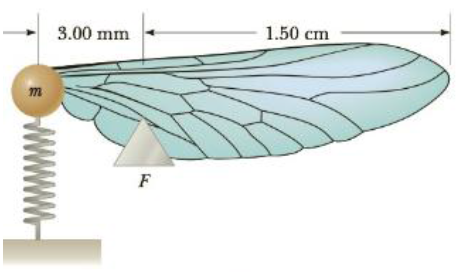Chapter 13, Problem 74AP

Chapter
Section
Textbook Problem

Figure P13.74 shows a crude model of an insect wing. The mass m represents the entire mass of the wing, which pivots about the fulcrum F. The spring represents the surrounding connective tissue. Motion of the wing corresponds to vibration of the spring. Suppose the mass of the wing is 0.30 g and the effective spring constant of the tissue is 4.7 ×10−4 N/m. If the mass m moves up and down a distance of 2.0 mm from its position of equilibrium, what is the maximum speed of the outer tip of the wing?Figure P13.74

To determine
The maximum speed of the outer tip of the wing.

Explanation

Given info: The spring constant of the spring is 4.7×104Nm-1 . The mass moves up and down a distance of 2.0mm about the equilibrium position. The mass of the entire wing is 0.30g .

From the conservation of total energy at equilibrium and turning position,

12kA2=12mvmax2

Solving for vmax ,

vmax=Akm

Substitute 4.7×104Nm-1 for k , 0.30g for m and 2.0mm for A to find out the maximum speed of the spring vmax

vmax=(2.0mm)4.7×104Nm-10.30g=(0.20cm)9.80Nm-10.30×103kg=0.25cms-1

Since the inner tip of the wing is attached to the spring, it will move with the same maximum speed like the spring.

Hence the maximum speed of the inner tip of the wing is 0.25cms-1 .

The total wing can be treated as rigid bar so all the points on the wing will rotate with the same angular velocity. The inner tip and the outer tip of the wing have different radii

Still sussing out bartleby?

Check out a sample textbook solution.

See a sample solution

The Solution to Your Study Problems

Bartleby provides explanations to thousands of textbook problems written by our experts, many with advanced degrees!

Get Started